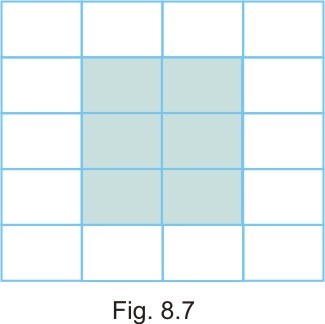#### In a floral design made from tiles each of dimensions 40cm by 60cm (See Fig. 8.7), find the ratios of: (a) the perimeter of shaded portion to the perimeter of the whole design. (b) the area of the shaded portion to the area of the unshaded portion.Length of one tile = 60 cm
The breadth of one tile =40 cm

Area of one tile =60 cm x 40 cm=2400 square cm
(a) The ratios of the perimeter of the shaded portion to the perimeter of the whole design.

(b) The ratios of the area of the shaded portion to the area of the unshaded portion